# NCERT Exemplar Solutions for Class 11 Maths Chapter 4 Principle of Mathematical Induction

NCERT Exemplar Solutions for Class 11 Maths Chapter 4 Principle of Mathematical Induction are useful for students, as they help them to score well in the exams. At BYJU’S, we aim to help students by providing detailed chapter-wise solutions to understand the concepts easily. The NCERT Exemplar Solutions contain detailed steps explaining all the problems that come under Chapter 4, Principle of Mathematical Induction of the Class 11 NCERT Exemplar Textbook. We have followed the latest syllabus while creating the NCERT Exemplar Solutions , and it is framed in accordance with the exam pattern of the CBSE Board. These solutions are designed by subject matter experts who have collated model questions covering all the exercise questions from the textbook. Apart from this, other resources are used to help students prepare for exams and score good marks. These resources include the NCERT notes, sample papers, textbooks, previous years’ papers, exemplar questions and so on.

In Chapter 4 of NCERT Exemplar Solutions for Class 11, students will learn and solve exemplar problems based on the chapter Principle of Mathematical Induction. If S(n) is a given statement, where n is a natural number, then

• The statement is true for n = 1, i.e., S(1) is true
• If the statement is true for n = k (where i is some positive integer), then the statement is also true for n = i + 1, i.e., the truth of S(k) implies the truth of S(i + 1).

Then, S(n) is true for all natural numbers n. The principle of mathematical induction is a technique used as proof in Mathematics. The major function of this principle is used as a defining property for every natural number, such as 1, 2, 3, 4, etc. The principle can effectively be proved with the help of two cases, which are the base case and the induction step. It is defined as the property which holds for the number 0. On the other hand, the property for one natural number is ‘n’, and the next natural number is ‘n+1’.

## Download the PDF of NCERT Exemplar Solutions for Class 11 Maths Chapter 4 – Principle of Mathematical Induction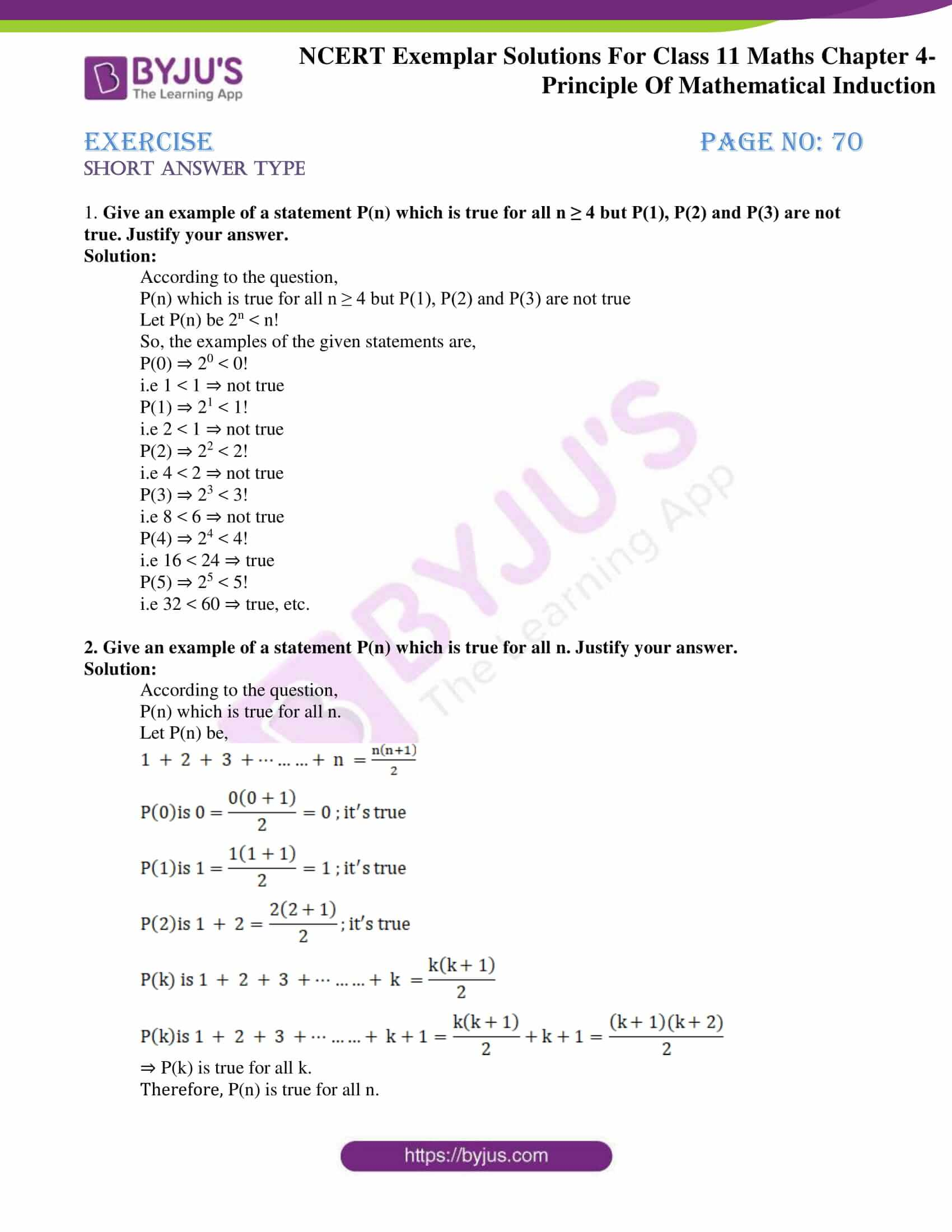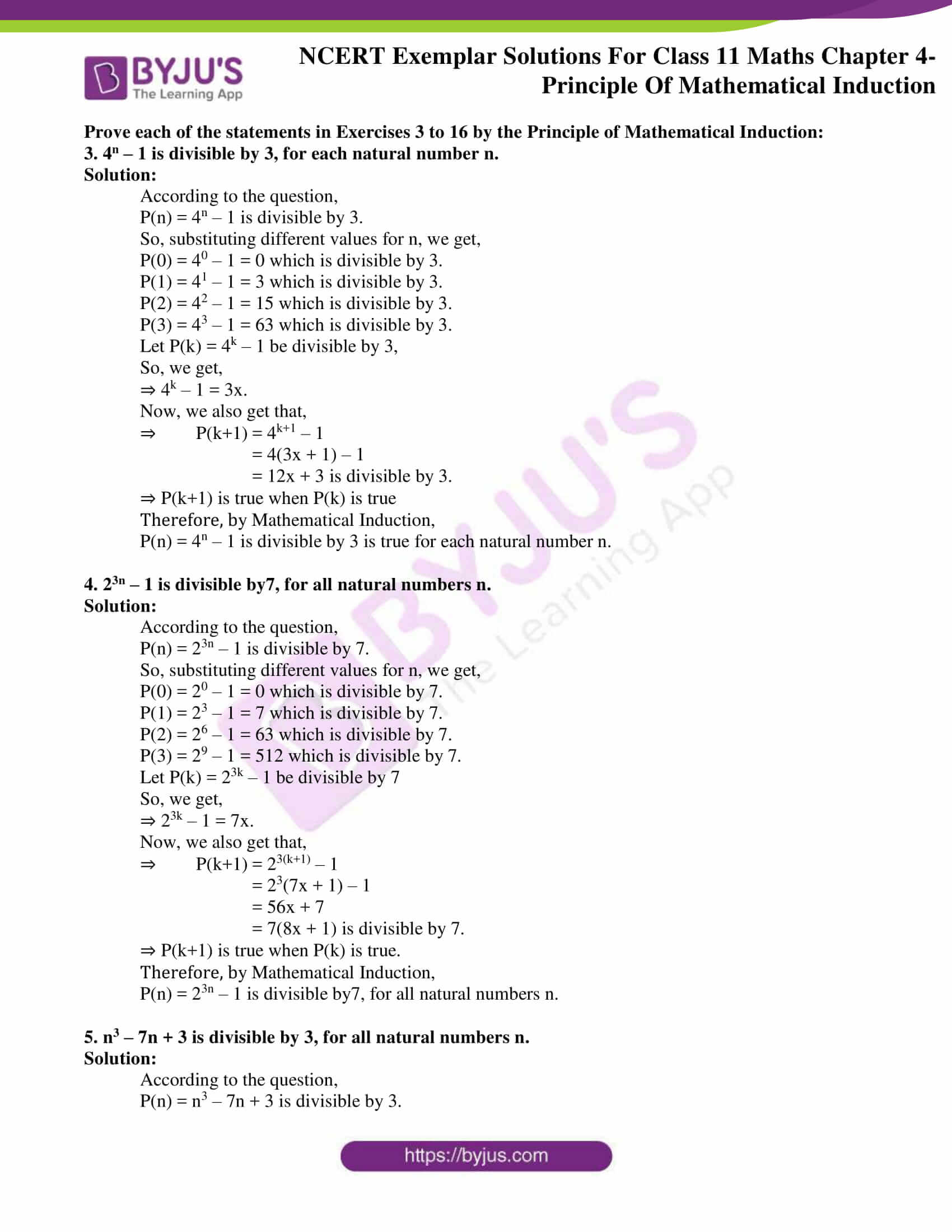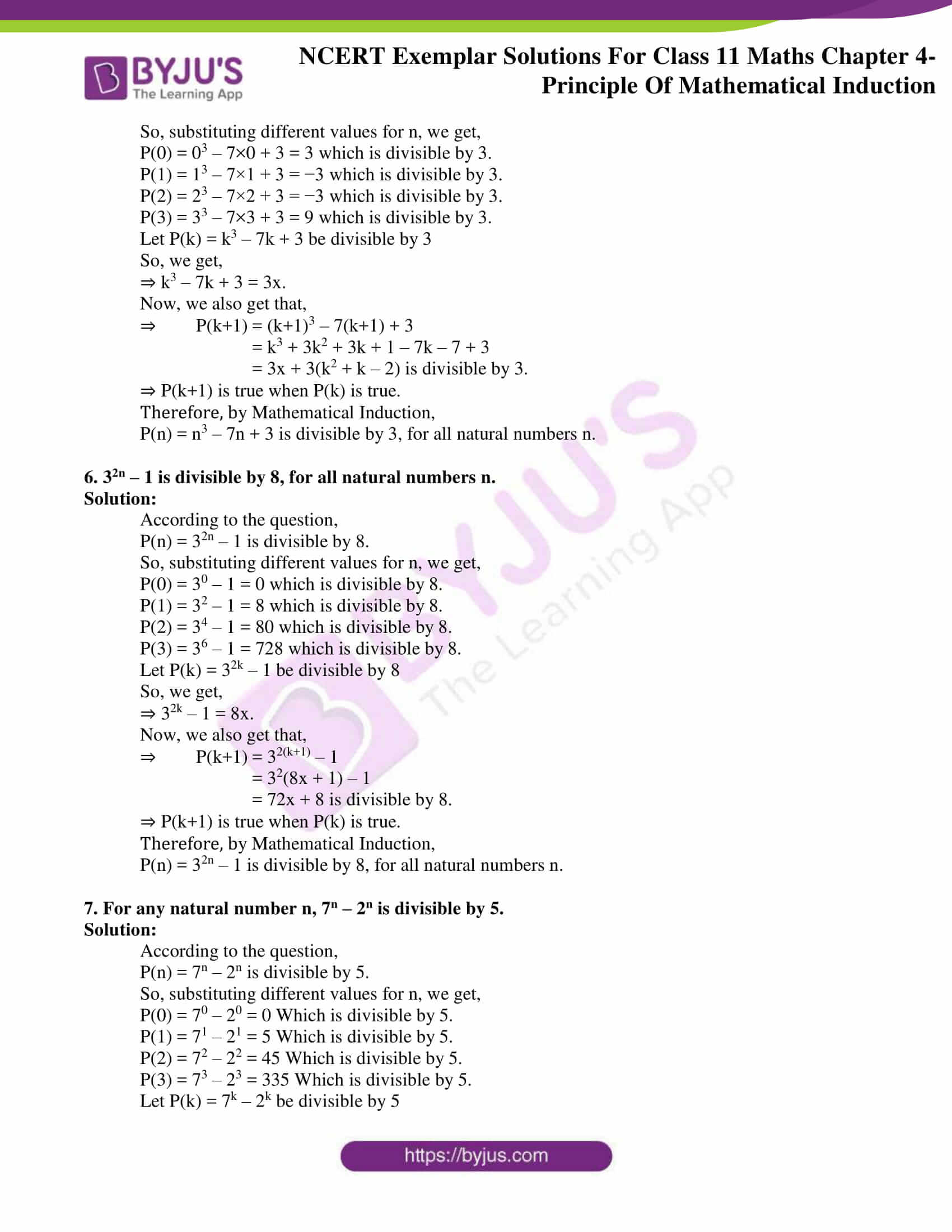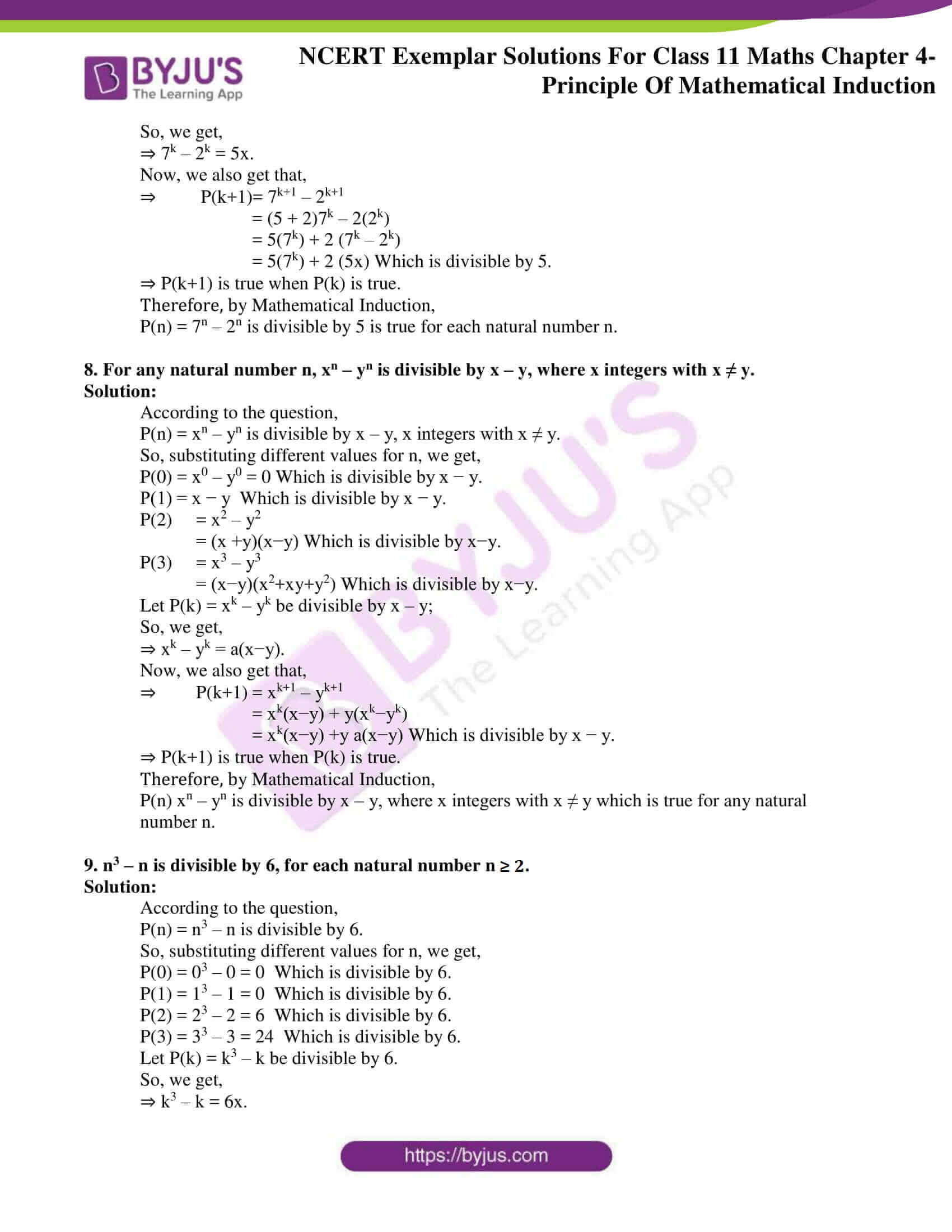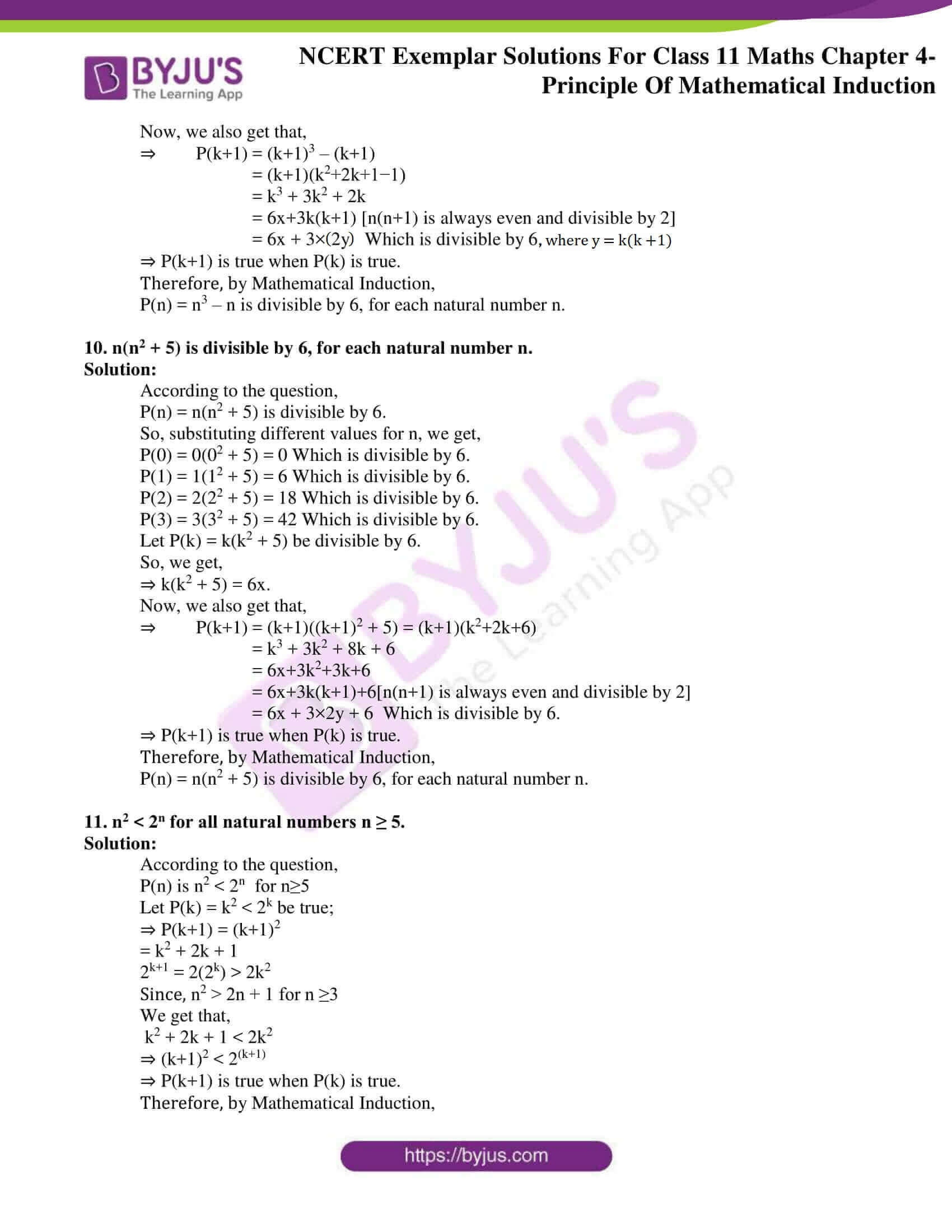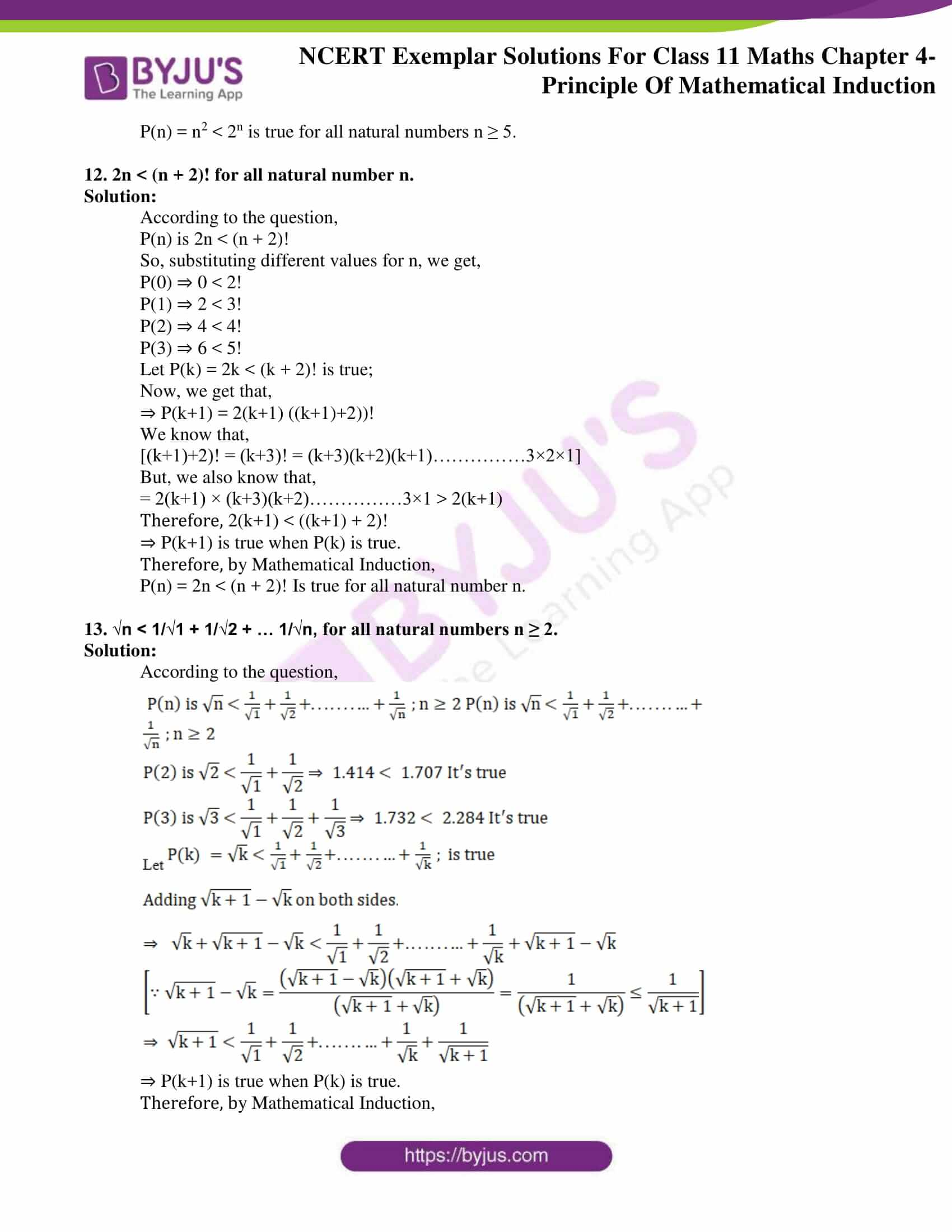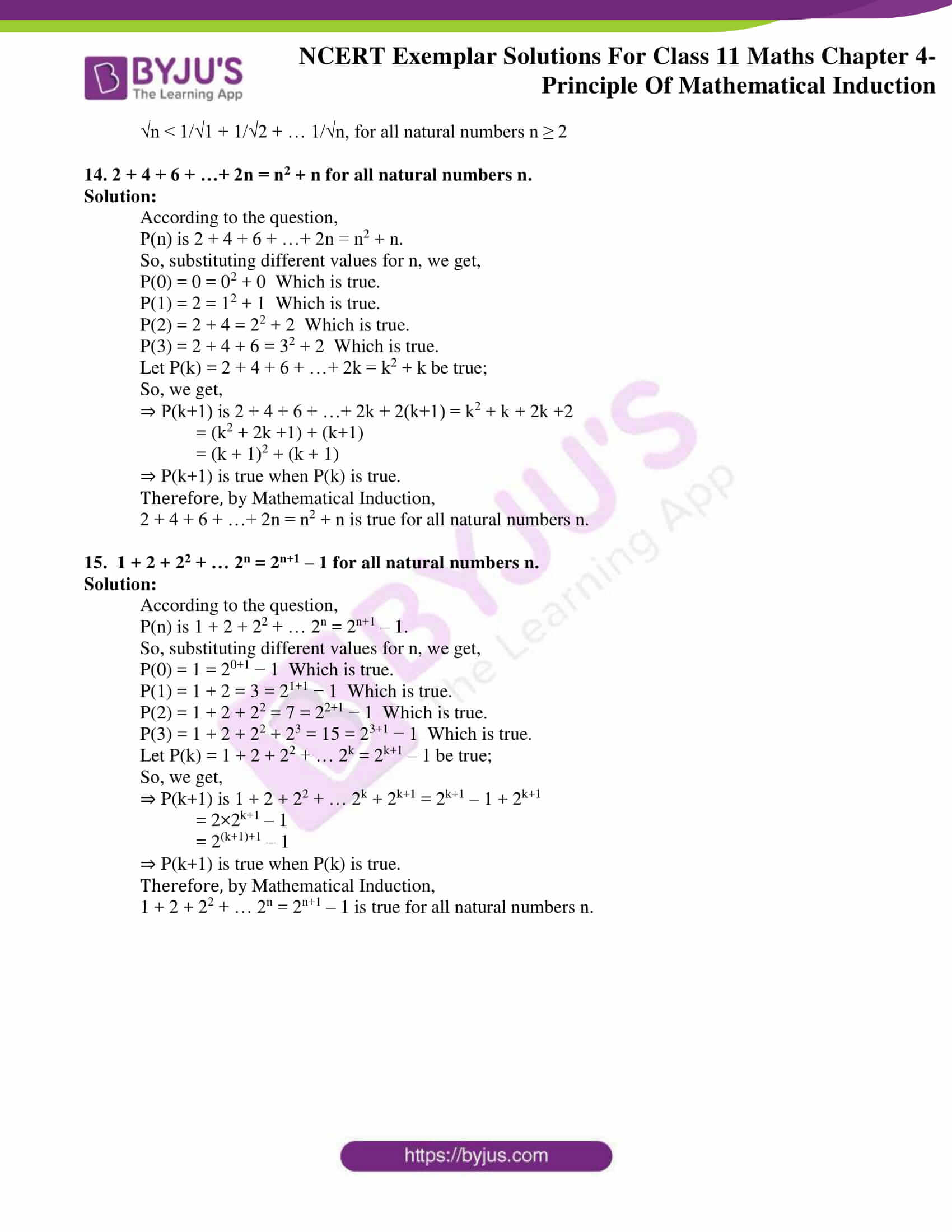### Access Answers to the NCERT Exemplar Class 11 Maths Chapter 4 – Principle of Mathematical Induction

Exercise Page No: 70

1. Give an example of a statement P(n) which is true for all n ≥ 4 but P(1), P(2) and P(3) are not true. Justify your answer.

Solution:

According to the question,

P(n) which is true for all n ≥ 4 but P(1), P(2) and P(3) are not true

Let P(n) be 2n < n!

So, the examples of the given statements are,

P(0) ⇒ 20 < 0!

i.e 1 < 1 ⇒ not true

P(1) ⇒ 21 < 1!

i.e 2 < 1 ⇒ not true

P(2) ⇒ 22 < 2!

i.e 4 < 2 ⇒ not true

P(3) ⇒ 23 < 3!

i.e 8 < 6 ⇒ not true

P(4) ⇒ 24 < 4!

i.e 16 < 24 ⇒ true

P(5) ⇒ 25 < 5!

i.e 32 < 60 ⇒ true, etc.

2. Give an example of a statement P(n) which is true for all n. Justify your answer.

Solution:

According to the question,

P(n) which is true for all n.

Let P(n) be,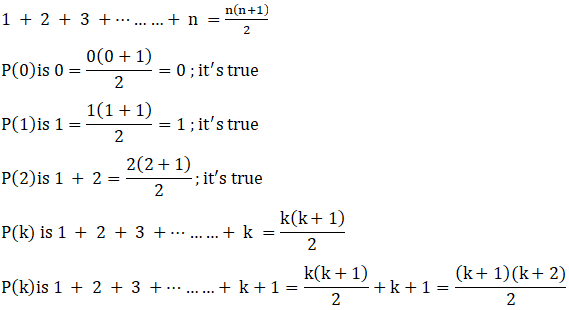⇒ P(k) is true for all k.

Therefore, P(n) is true for all n.

Prove each of the statements in Exercises 3 to 16 by the Principle of Mathematical Induction:

3. 4n – 1 is divisible by 3, for each natural number n.

Solution:

According to the question,

P(n) = 4n – 1 is divisible by 3.

So, substituting different values for n, we get,

P(0) = 40 – 1 = 0 which is divisible by 3.

P(1) = 41 – 1 = 3 which is divisible by 3.

P(2) = 42 – 1 = 15 which is divisible by 3.

P(3) = 43 – 1 = 63 which is divisible by 3.

Let P(k) = 4k – 1 be divisible by 3,

So, we get,

⇒ 4k – 1 = 3x.

Now, we also get that,

⇒  P(k+1) = 4k+1 – 1

= 4(3x + 1) – 1

= 12x + 3 is divisible by 3.

⇒ P(k+1) is true when P(k) is true

Therefore, by Mathematical Induction,

P(n) = 4n – 1 is divisible by 3 is true for each natural number n.

4. 23n – 1 is divisible by7, for all natural numbers n.

Solution:

According to the question,

P(n) = 23n – 1 is divisible by 7.

So, substituting different values for n, we get,

P(0) = 20 – 1 = 0 which is divisible by 7.

P(1) = 23 – 1 = 7 which is divisible by 7.

P(2) = 26 – 1 = 63 which is divisible by 7.

P(3) = 29 – 1 = 512 which is divisible by 7.

Let P(k) = 23k – 1 be divisible by 7

So, we get,

⇒ 23k – 1 = 7x.

Now, we also get that,

⇒  P(k+1) = 23(k+1) – 1

= 23(7x + 1) – 1

= 56x + 7

= 7(8x + 1) is divisible by 7.

⇒ P(k+1) is true when P(k) is true.

Therefore, by Mathematical Induction,

P(n) = 23n – 1 is divisible by7, for all natural numbers n.

5. n3 – 7n + 3 is divisible by 3, for all natural numbers n.

Solution:

According to the question,

P(n) = n3 – 7n + 3 is divisible by 3.

So, substituting different values for n, we get,

P(0) = 03 – 7×0 + 3 = 3 which is divisible by 3.

P(1) = 13 – 7×1 + 3 = −3 which is divisible by 3.

P(2) = 23 – 7×2 + 3 = −3 which is divisible by 3.

P(3) = 33 – 7×3 + 3 = 9 which is divisible by 3.

Let P(k) = k3 – 7k + 3 be divisible by 3

So, we get,

⇒ k3 – 7k + 3 = 3x.

Now, we also get that,

⇒  P(k+1) = (k+1)3 – 7(k+1) + 3

= k3 + 3k2 + 3k + 1 – 7k – 7 + 3

= 3x + 3(k2 + k – 2) is divisible by 3.

⇒ P(k+1) is true when P(k) is true.

Therefore, by Mathematical Induction,

P(n) = n3 – 7n + 3 is divisible by 3, for all natural numbers n.

6. 32n – 1 is divisible by 8, for all natural numbers n.

Solution:

According to the question,

P(n) = 32n – 1 is divisible by 8.

So, substituting different values for n, we get,

P(0) = 30 – 1 = 0 which is divisible by 8.

P(1) = 32 – 1 = 8 which is divisible by 8.

P(2) = 34 – 1 = 80 which is divisible by 8.

P(3) = 36 – 1 = 728 which is divisible by 8.

Let P(k) = 32k – 1 be divisible by 8

So, we get,

⇒ 32k – 1 = 8x.

Now, we also get that,

⇒  P(k+1) = 32(k+1) – 1

= 32(8x + 1) – 1

= 72x + 8 is divisible by 8.

⇒ P(k+1) is true when P(k) is true.

Therefore, by Mathematical Induction,

P(n) = 32n – 1 is divisible by 8, for all natural numbers n.

7. For any natural number n, 7n – 2n is divisible by 5.

Solution:

According to the question,

P(n) = 7n – 2n is divisible by 5.

So, substituting different values for n, we get,

P(0) = 70 – 20 = 0 Which is divisible by 5.

P(1) = 71 – 21 = 5 Which is divisible by 5.

P(2) = 72 – 22 = 45 Which is divisible by 5.

P(3) = 73 – 23 = 335 Which is divisible by 5.

Let P(k) = 7k – 2k be divisible by 5

So, we get,

⇒ 7k – 2k = 5x.

Now, we also get that,

⇒  P(k+1)= 7k+1 – 2k+1

= (5 + 2)7k – 2(2k)

= 5(7k) + 2 (7k – 2k)

= 5(7k) + 2 (5x) Which is divisible by 5.

⇒ P(k+1) is true when P(k) is true.

Therefore, by Mathematical Induction,

P(n) = 7n – 2n is divisible by 5 is true for each natural number n.

8. For any natural number n, xn – yn is divisible by x – y, where x integers with x ≠ y.

Solution:

According to the question,

P(n) = xn – yn is divisible by x – y, x integers with x ≠ y.

So, substituting different values for n, we get,

P(0) = x0 – y0 = 0 Which is divisible by x − y.

P(1) = x − y Which is divisible by x − y.

P(2) = x2 – y2

= (x +y)(x−y) Which is divisible by x−y.

P(3) = x3 – y3

= (x−y)(x2+xy+y2) Which is divisible by x−y.

Let P(k) = xk – yk be divisible by x – y;

So, we get,

⇒ xk – yk = a(x−y).

Now, we also get that,

⇒  P(k+1) = xk+1 – yk+1

= xk(x−y) + y(xk−yk)

= xk(x−y) +y a(x−y) Which is divisible by x − y.

⇒ P(k+1) is true when P(k) is true.

Therefore, by Mathematical Induction,

P(n) xn – yn is divisible by x – y, where x integers with x ≠ y which is true for any natural number n.

9. n3 – n is divisible by 6, for each natural number n ≥ 2.

Solution:

According to the question,

P(n) = n3 – n is divisible by 6.

So, substituting different values for n, we get,

P(0) = 03 – 0 = 0 Which is divisible by 6.

P(1) = 13 – 1 = 0 Which is divisible by 6.

P(2) = 23 – 2 = 6 Which is divisible by 6.

P(3) = 33 – 3 = 24 Which is divisible by 6.

Let P(k) = k3 – k be divisible by 6.

So, we get,

⇒ k3 – k = 6x.

Now, we also get that,

⇒  P(k+1) = (k+1)3 – (k+1)

= (k+1)(k2+2k+1−1)

= k3 + 3k2 + 2k

= 6x+3k(k+1) [n(n+1) is always even and divisible by 2]

= 6x + 3×(2y) Which is divisible by 6, where y = k(k+1)

⇒ P(k+1) is true when P(k) is true.

Therefore, by Mathematical Induction,

P(n) = n3 – n is divisible by 6, for each natural number n.

10. n(n2 + 5) is divisible by 6, for each natural number n.

Solution:

According to the question,

P(n) = n(n2 + 5) is divisible by 6.

So, substituting different values for n, we get,

P(0) = 0(02 + 5) = 0 Which is divisible by 6.

P(1) = 1(12 + 5) = 6 Which is divisible by 6.

P(2) = 2(22 + 5) = 18 Which is divisible by 6.

P(3) = 3(32 + 5) = 42 Which is divisible by 6.

Let P(k) = k(k2 + 5) be divisible by 6.

So, we get,

⇒ k(k2 + 5) = 6x.

Now, we also get that,

⇒  P(k+1) = (k+1)((k+1)2 + 5) = (k+1)(k2+2k+6)

= k3 + 3k2 + 8k + 6

= 6x+3k2+3k+6

= 6x+3k(k+1)+6[n(n+1) is always even and divisible by 2]

= 6x + 3×2y + 6 Which is divisible by 6.

⇒ P(k+1) is true when P(k) is true.

Therefore, by Mathematical Induction,

P(n) = n(n2 + 5) is divisible by 6, for each natural number n.

11. n2 < 2n for all natural numbers n ≥ 5.

Solution:

According to the question,

P(n) is n2 < 2n  for n≥5

Let P(k) = k2 < 2k be true;

⇒ P(k+1) = (k+1)2

= k2 + 2k + 1

2k+1 = 2(2k) > 2k2

Since, n2 > 2n + 1 for n ≥3

We get that,

k2 + 2k + 1 < 2k2

⇒ (k+1)2 < 2(k+1)

⇒ P(k+1) is true when P(k) is true.

Therefore, by Mathematical Induction,

P(n) = n2 < 2n is true for all natural numbers n ≥ 5.

12. 2n < (n + 2)! for all natural number n.

Solution:

According to the question,

P(n) is 2n < (n + 2)!

So, substituting different values for n, we get,

P(0) ⇒ 0 < 2!

P(1) ⇒ 2 < 3!

P(2) ⇒ 4 < 4!

P(3) ⇒ 6 < 5!

Let P(k) = 2k < (k + 2)! is true;

Now, we get that,

⇒ P(k+1) = 2(k+1) ((k+1)+2))!

We know that,

[(k+1)+2)! = (k+3)! = (k+3)(k+2)(k+1)……………3×2×1]

But, we also know that,

= 2(k+1) × (k+3)(k+2)……………3×1 > 2(k+1)

Therefore, 2(k+1) < ((k+1) + 2)!

⇒ P(k+1) is true when P(k) is true.

Therefore, by Mathematical Induction,

P(n) = 2n < (n + 2)! Is true for all natural number n.

13. √n < 1/√1 + 1/√2 + … 1/√n, for all natural numbers n ≥ 2.

Solution:

According to the question,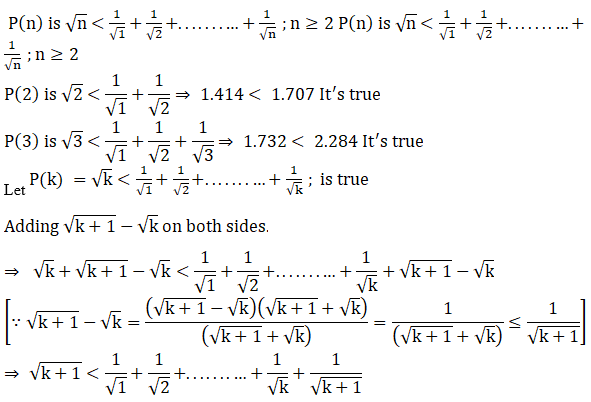⇒ P(k+1) is true when P(k) is true.

Therefore, by Mathematical Induction,

√n < 1/√1 + 1/√2 + … 1/√n, for all natural numbers n ≥ 2

14. 2 + 4 + 6 + …+ 2n = n2 + n for all natural numbers n.

Solution:

According to the question,

P(n) is 2 + 4 + 6 + …+ 2n = n2 + n.

So, substituting different values for n, we get,

P(0) = 0 = 02 + 0 Which is true.

P(1) = 2 = 12 + 1 Which is true.

P(2) = 2 + 4 = 22 + 2 Which is true.

P(3) = 2 + 4 + 6 = 32 + 2 Which is true.

Let P(k) = 2 + 4 + 6 + …+ 2k = k2 + k be true;

So, we get,

⇒ P(k+1) is 2 + 4 + 6 + …+ 2k + 2(k+1) = k2 + k + 2k +2

= (k2 + 2k +1) + (k+1)

= (k + 1)2 + (k + 1)

⇒ P(k+1) is true when P(k) is true.

Therefore, by Mathematical Induction,

2 + 4 + 6 + …+ 2n = n2 + n is true for all natural numbers n.

15. 1 + 2 + 22 + … 2n = 2n+1 – 1 for all natural numbers n.

Solution:

According to the question,

P(n) is 1 + 2 + 22 + … 2n = 2n+1 – 1.

So, substituting different values for n, we get,

P(0) = 1 = 20+1 − 1 Which is true.

P(1) = 1 + 2 = 3 = 21+1 − 1 Which is true.

P(2) = 1 + 2 + 22 = 7 = 22+1 − 1 Which is true.

P(3) = 1 + 2 + 22 + 23 = 15 = 23+1 − 1 Which is true.

Let P(k) = 1 + 2 + 22 + … 2k = 2k+1 – 1 be true;

So, we get,

⇒ P(k+1) is 1 + 2 + 22 + … 2k + 2k+1 = 2k+1 – 1 + 2k+1

= 2×2k+1 – 1

= 2(k+1)+1 – 1

⇒ P(k+1) is true when P(k) is true.

Therefore, by Mathematical Induction,

1 + 2 + 22 + … 2n = 2n+1 – 1 is true for all natural numbers n.